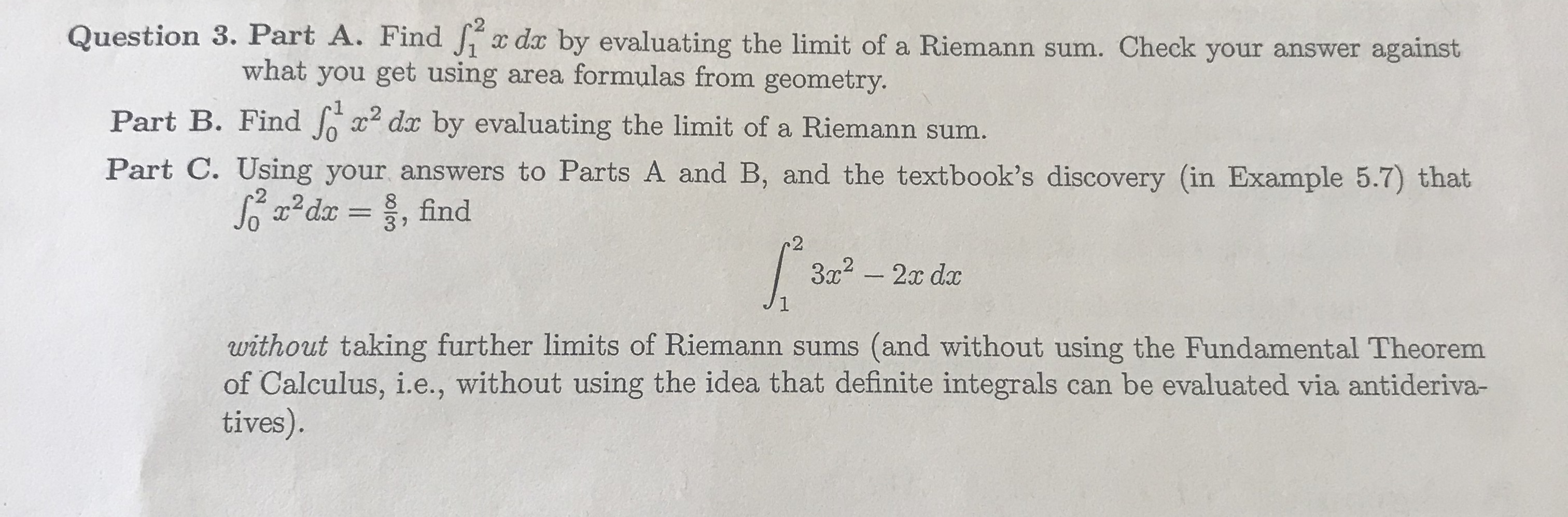# Question 3. Part A. Findda by evaluating the limit of a Riemann sum. Check your answer againstwhat you get using area formulas from geometryPart B. Find Jo 2 da by evaluating the limit of a Riemann sum.Part C. Using your answers to Parts A and B, and the textbook's discovery (in Example 5.7) that03 72without taking further limits of Riemann sums (and without using the Fundamental Theoremof Calculus, i.e., without using the idea that definite integrals can be evaluated via antideriva-tives).

Question
57 views

How would you solve for 3help_outlineImage TranscriptioncloseQuestion 3. Part A. Find da by evaluating the limit of a Riemann sum. Check your answer against what you get using area formulas from geometry Part B. Find Jo 2 da by evaluating the limit of a Riemann sum. Part C. Using your answers to Parts A and B, and the textbook's discovery (in Example 5.7) that 0 3 7 2 without taking further limits of Riemann sums (and without using the Fundamental Theorem of Calculus, i.e., without using the idea that definite integrals can be evaluated via antideriva- tives). fullscreen
check_circle

Step 1

To calculate the provided definite integral by using the result that was calculated in Part A. and Part B. The value of the integral in Part A. and Part B was calculated by Riemann sum. Now, first convert the provided definite integral in Part C in the form of Part A. and Part B.

Step 2

According to the formula of Riemann sum by limit is shown below,

Step 3

Now, calculate the value of Part A. by Riemann sum and the provided definit...

### Want to see the full answer?

See Solution

#### Want to see this answer and more?

Solutions are written by subject experts who are available 24/7. Questions are typically answered within 1 hour.*

See Solution
*Response times may vary by subject and question.
Tagged in

### Calculus